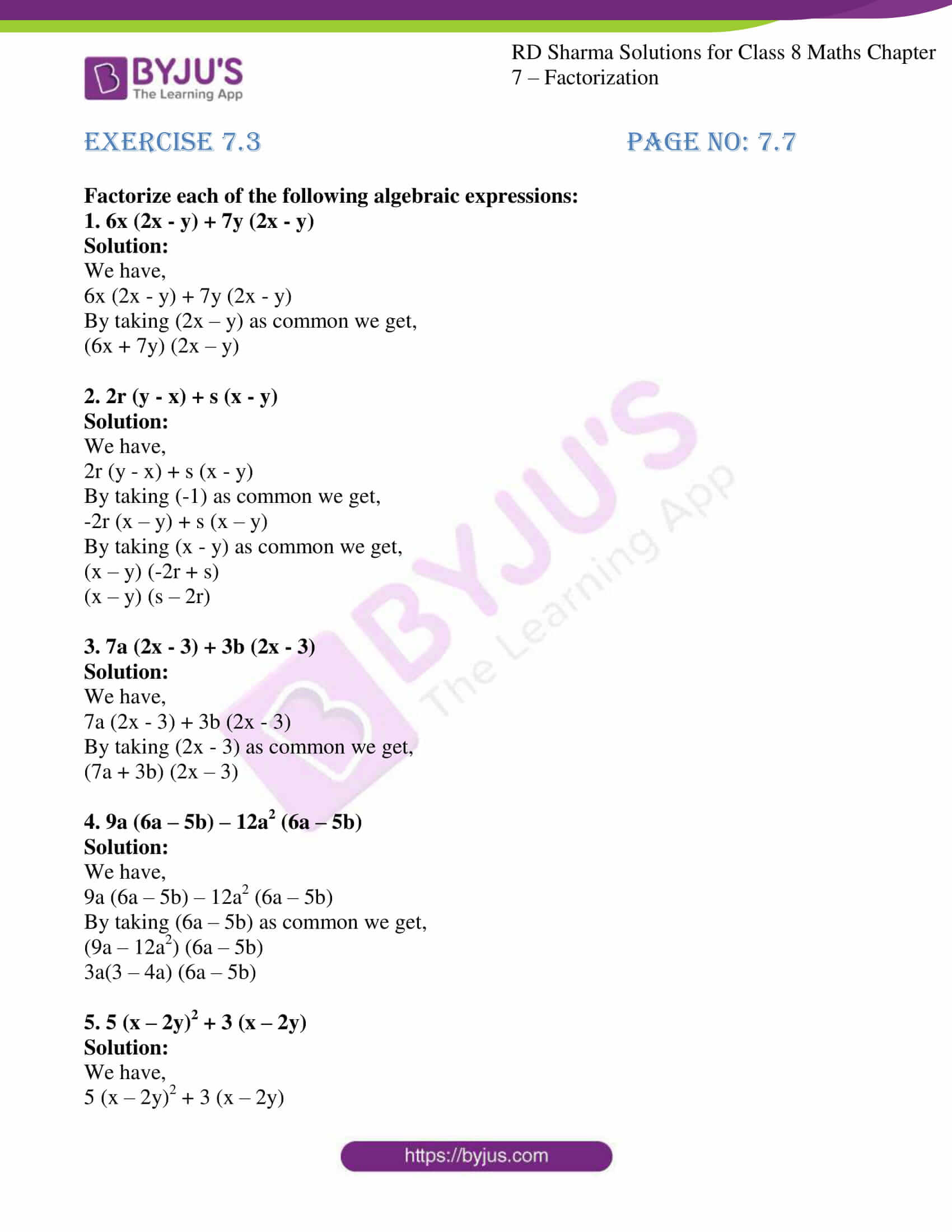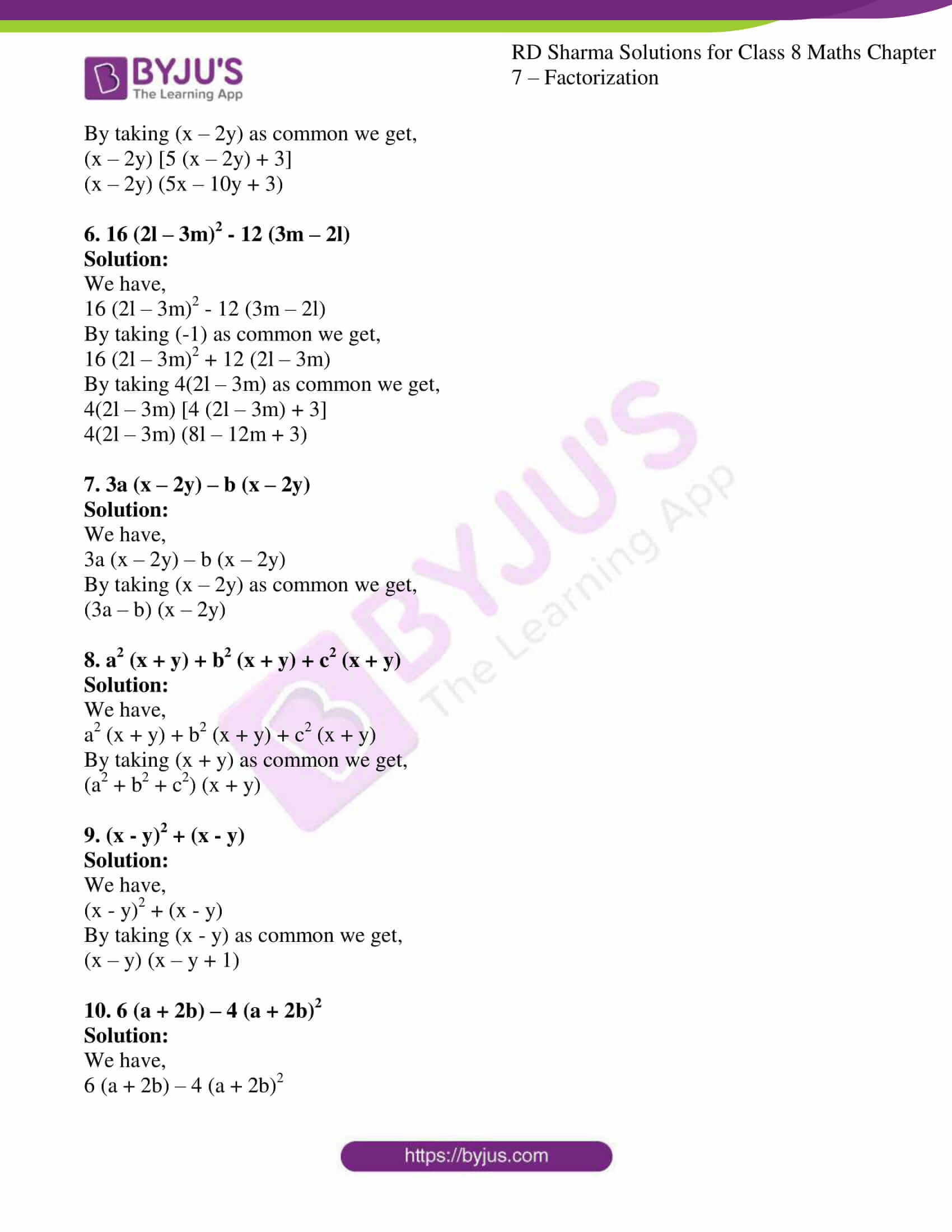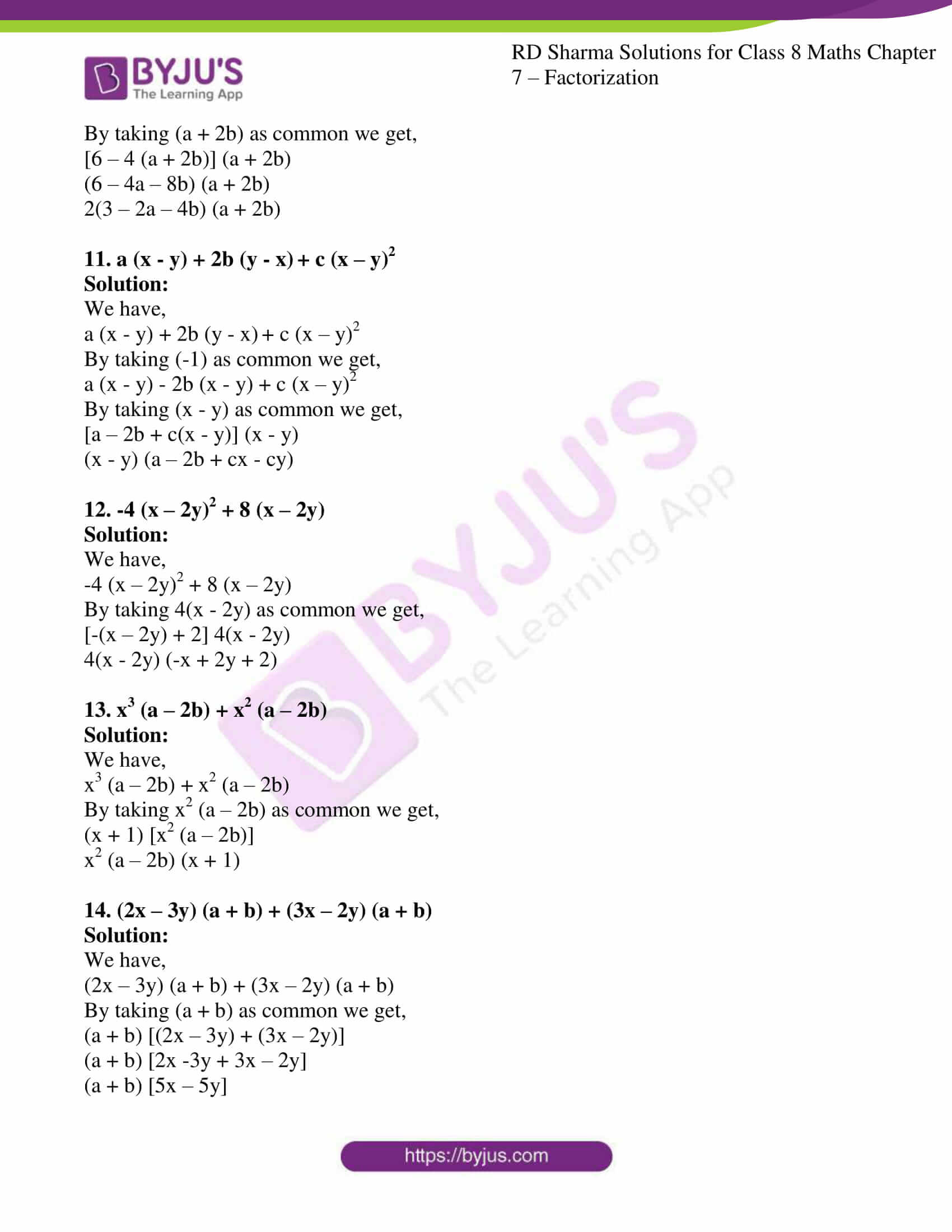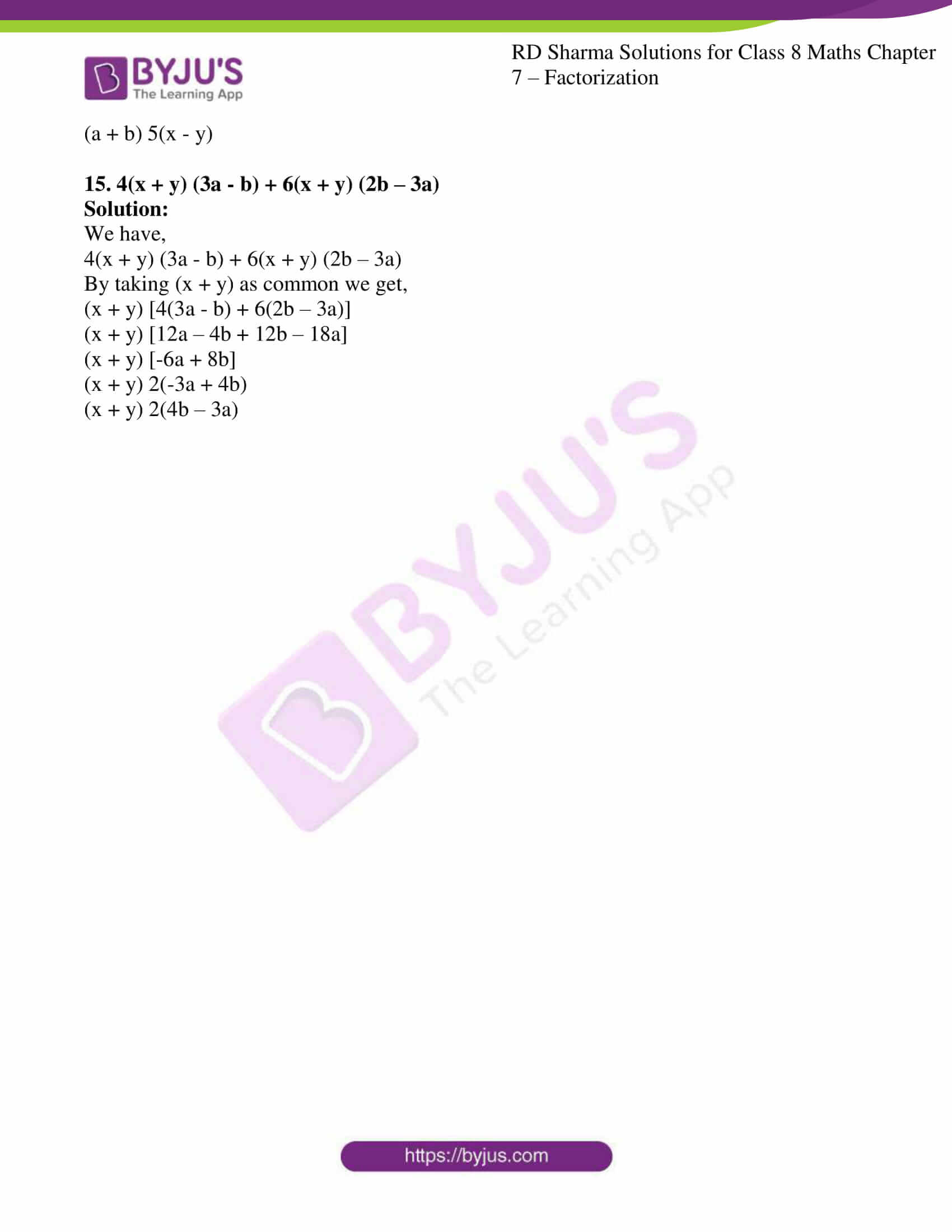# RD Sharma Solutions for Class 8 Maths Chapter - 7 Factorization Exercise 7.3

Students can refer to RD Sharma Solutions for Class 8 Maths Exercise 7.3 Chapter 7 Factorization which are available here. The solutions here are solved step by step for a better understanding of the concepts, which helps students prepare for their exams at ease. Keeping in mind expert tutors at BYJU’S have made this possible to help students crack difficult problems. Students can download the pdf of RD Sharma Solutions from the links provided below. Exercise 7.3 of Chapter 7 Factorization is on based on the factorization of algebraic expressions when a binomial is a common factor.

## Download the pdf of RD Sharma Solutions For Class 8 Maths Exercise 7.3 Chapter 7 Factorization### Access Answers to RD Sharma Solutions for Class 8 Maths Exercise 7.3 Chapter 7 Factorization

#### EXERCISE 7.3 PAGE NO: 7.7

Factorize each of the following algebraic expressions:

1. 6x (2x – y) + 7y (2x – y)

Solution:

We have,

6x (2x – y) + 7y (2x – y)

By taking (2x – y) as common we get,

(6x + 7y) (2x – y)

2. 2r (y – x) + s (x – y)

Solution:

We have,

2r (y – x) + s (x – y)

By taking (-1) as common we get,

-2r (x – y) + s (x – y)

By taking (x – y) as common we get,

(x – y) (-2r + s)

(x – y) (s – 2r)

3. 7a (2x – 3) + 3b (2x – 3)

Solution:

We have,

7a (2x – 3) + 3b (2x – 3)

By taking (2x – 3) as common we get,

(7a + 3b) (2x – 3)

4. 9a (6a – 5b) – 12a2 (6a – 5b)

Solution:

We have,

9a (6a – 5b) – 12a2 (6a – 5b)

By taking (6a – 5b) as common we get,

(9a – 12a2) (6a – 5b)

3a(3 – 4a) (6a – 5b)

5. 5 (x – 2y)2 + 3 (x – 2y)

Solution:

We have,

5 (x – 2y)2 + 3 (x – 2y)

By taking (x – 2y) as common we get,

(x – 2y) [5 (x – 2y) + 3]

(x – 2y) (5x – 10y + 3)

6. 16 (2l – 3m)2 – 12 (3m – 2l)

Solution:

We have,

16 (2l – 3m)2 – 12 (3m – 2l)

By taking (-1) as common we get,

16 (2l – 3m)2 + 12 (2l – 3m)

By taking 4(2l – 3m) as common we get,

4(2l – 3m) [4 (2l – 3m) + 3]

4(2l – 3m) (8l – 12m + 3)

7. 3a (x – 2y) – b (x – 2y)

Solution:

We have,

3a (x – 2y) – b (x – 2y)

By taking (x – 2y) as common we get,

(3a – b) (x – 2y)

8. a2 (x + y) + b2 (x + y) + c2 (x + y)

Solution:

We have,

a2 (x + y) + b2 (x + y) + c2 (x + y)

By taking (x + y) as common we get,

(a2 + b2 + c2) (x + y)

9. (x – y)2 + (x – y)

Solution:

We have,

(x – y)2 + (x – y)

By taking (x – y) as common we get,

(x – y) (x – y + 1)

10. 6 (a + 2b) – 4 (a + 2b)2

Solution:

We have,

6 (a + 2b) – 4 (a + 2b)2

By taking (a + 2b) as common we get,

[6 – 4 (a + 2b)] (a + 2b)

(6 – 4a – 8b) (a + 2b)

2(3 – 2a – 4b) (a + 2b)

11. a (x – y) + 2b (y – x) + c (x – y)2

Solution:

We have,

a (x – y) + 2b (y – x) + c (x – y)2

By taking (-1) as common we get,

a (x – y) – 2b (x – y) + c (x – y)2

By taking (x – y) as common we get,

[a – 2b + c(x – y)] (x – y)

(x – y) (a – 2b + cx – cy)

12. -4 (x – 2y)2 + 8 (x – 2y)

Solution:

We have,

-4 (x – 2y)2 + 8 (x – 2y)

By taking 4(x – 2y) as common we get,

[-(x – 2y) + 2] 4(x – 2y)

4(x – 2y) (-x + 2y + 2)

13. x3 (a – 2b) + x2 (a – 2b)

Solution:

We have,

x3 (a – 2b) + x2 (a – 2b)

By taking x2 (a – 2b) as common we get,

(x + 1) [x2 (a – 2b)]

x2 (a – 2b) (x + 1)

14. (2x – 3y) (a + b) + (3x – 2y) (a + b)

Solution:

We have,

(2x – 3y) (a + b) + (3x – 2y) (a + b)

By taking (a + b) as common we get,

(a + b) [(2x – 3y) + (3x – 2y)]

(a + b) [2x -3y + 3x – 2y]

(a + b) [5x – 5y]

(a + b) 5(x – y)

15. 4(x + y) (3a – b) + 6(x + y) (2b – 3a)

Solution:

We have,

4(x + y) (3a – b) + 6(x + y) (2b – 3a)

By taking (x + y) as common we get,

(x + y) [4(3a – b) + 6(2b – 3a)]

(x + y) [12a – 4b + 12b – 18a]

(x + y) [-6a + 8b]

(x + y) 2(-3a + 4b)

(x + y) 2(4b – 3a)